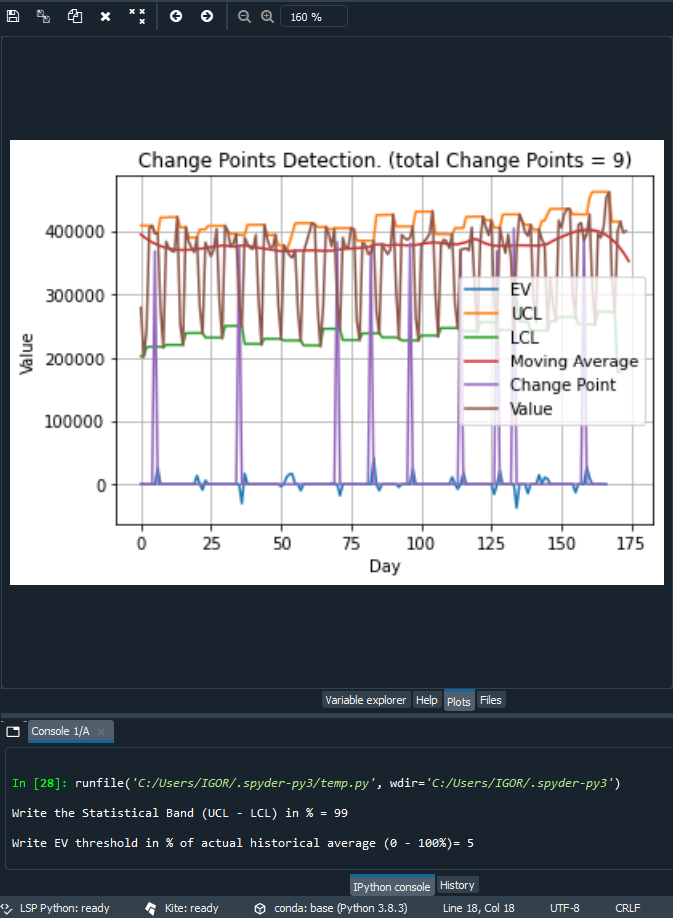## Saturday, August 22, 2020

### CPD - Change Points Detection is planed to be implemented in the free web tool Perfomalist

The next version of the Perfomalist (https://www.perfomalist.com/ ) is coming and will include a new functionality  - Change Point Detection.

How to find a change in the historical time-series data?

Long ago I have developed a method to do that which is based on EV data (Exception Value - a magnitude of anomalies collected historically). Idea: any change occurred  first would appear as  anomaly and then become a normality (norm), so collecting and analyzing a severity of all anomalies opens possibility to find phases in the history with different patterns. To detect that mathematically one needs just finds all roots of the following equation:   EV(t)=0 , where t is time. But it is too simple as that might give you too many change points. To control the the sensitivity of detecting change points the method  should have some sensitivity tuning parameters,such as following:

Nnormality confidence band in percentiles = UCL-LCL (if it is 100%, that means all observations is normal, 0% means all observations abnormal)

Imodel insensitivity = EV threshold (if it is 0, that means maximum sensitivity which gives the maximum change points for the given confidence band).

Respectively the more accurate model would be defined by  the following equation:

|EV(t,N)|=I  or

|EV(t,UCL-LCL)|=I

Where UCL is upper control limit and LCL is lower control limit. Why "||" (absolute value)? To catch two types of changes:  going up- and downwards.

How the EV(t) function is defined explained in the following white paper -

### (PDF) Exception Based Modeling and Forecasting.

This CPD method has been coded by one of the Performalist developer as a python program and here is a test result:Currently the approach has been testing and  the respectful  micro-service (API) has been developing.  Stay tuned!